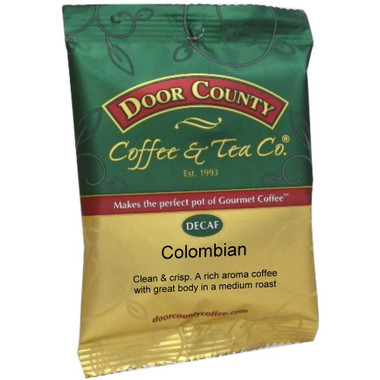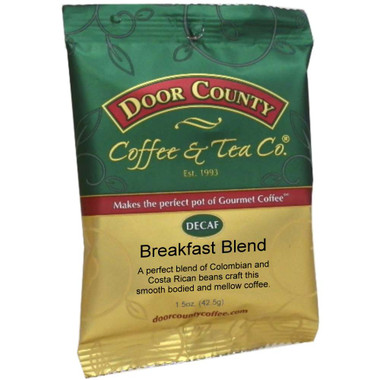•Quantity Decrease Quantity Increase Quantity Decrease Quantity
•Quantity Decrease Quantity Increase Quantity Decrease Quantity
•Quantity Decrease Quantity Increase Quantity Decrease Quantity
•Quantity Decrease Quantity Increase Quantity Decrease Quantity
\$54.99
\$59.99
•Quantity Decrease Quantity Increase Quantity Decrease Quantity
\$54.99
\$59.99
•Quantity Decrease Quantity Increase Quantity Decrease Quantity
\$54.99
\$59.99
•Quantity Decrease Quantity Increase Quantity Decrease Quantity
\$54.99
\$59.99
•Quantity Decrease Quantity Increase Quantity Decrease Quantity
\$54.99
\$59.99
•Quantity Decrease Quantity Increase Quantity Decrease Quantity
\$54.99
\$59.99
•Quantity Decrease Quantity Increase Quantity Decrease Quantity
•Quantity Decrease Quantity Increase Quantity Decrease Quantity
•Quantity Decrease Quantity Increase Quantity Decrease Quantity